Surfaces of revolution • from [Kü03] pp.52-54

We walk thru the computations carried out on pages 53-54 in [Kü03] to compare the results.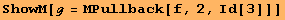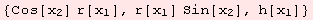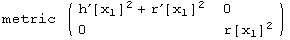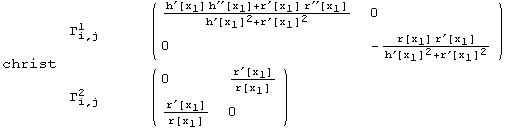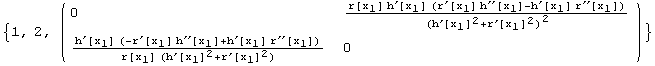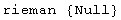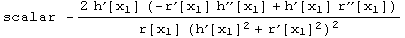We make the same assumptions as [Kü03].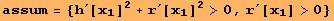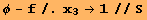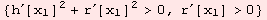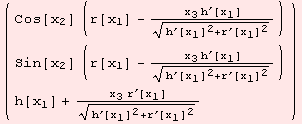All figures below are labelled. Without additional assumptions, the geometrical expressions get quite complicated.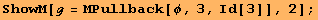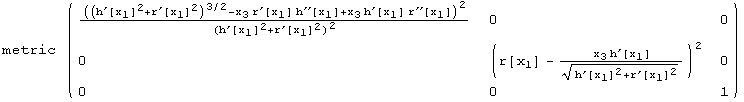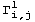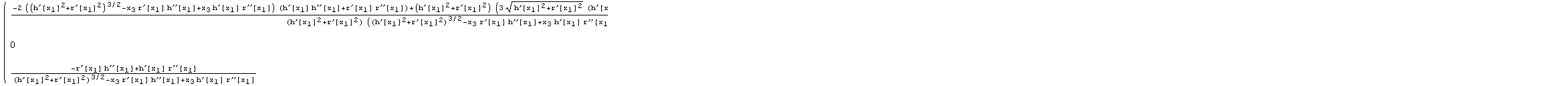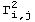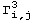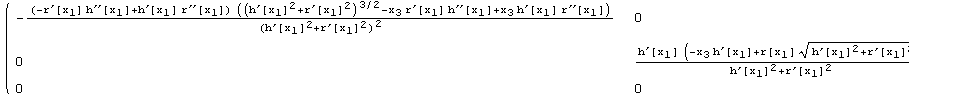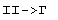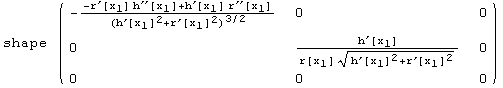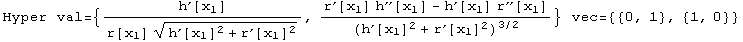To simplify the discussion, at this point [Kü03] makes the stronger assuptions that the curve (r,h) is parametrised according to arc length.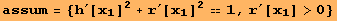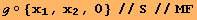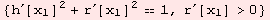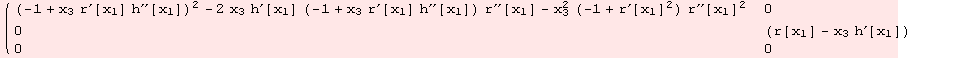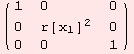All expressions are labelled: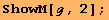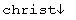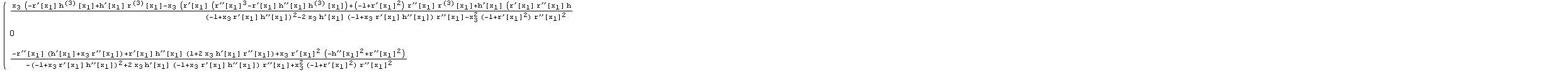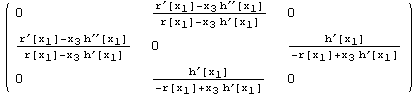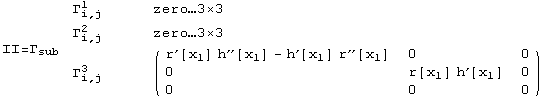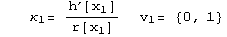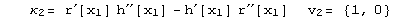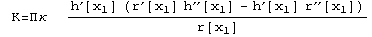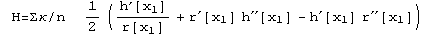Created by Mathematica  (December 22, 2006)